# H^infinity-control-theory

A branch of control theory where the performance of a dynamical system (cf. Automatic control theory) is appraised in terms of the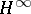-norm. The Banach space(named after G.H. Hardy, cf. Hardy classes) consists of all complex-valued functions of a complex variable which are analytic and of bounded modulus in the open right half-plane. The norm of such a function is the supremum modulus: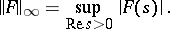By a theorem of Fatou (cf. Fatou theorem), such a function has a boundary valuefor almost-all, and, moreover,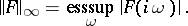The theory ofcontrol was initiated by G. Zames [a1], [a2], [a3], who formulated a basic feedback problem as an optimization problem with an operator norm, in particular, an-norm. Relevant contemporaneous works are those of J.W. Helton [a4] and A. Tannenbaum [a5].

The theory treats dynamical systems represented as integral operators of the form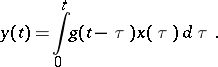Hereis sufficiently regular to make the input-output mapping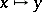a bounded operator on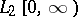. Taking Laplace transforms gives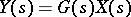. The functionis called the transfer function of the system and it belongs tobecause the integral operator is bounded. Moreover, the-norm ofequals the norm of the integral operator, i.e.,(a1)

There are two prototype problems giving rise to an optimality criterion with the-norm. The first is the problem of robust stability of the feedback system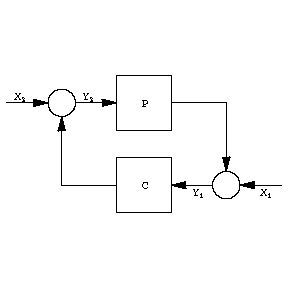Figure: h046020a

Hereandare transfer functions in, and,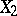,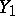,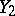are Laplace transforms of signals;represents a "plant" , the dynamical system which is to be controlled, andrepresents the "controller" (cf. also Automatic control theory). The figure stands for the two equations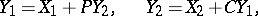which can be solved to giveTherefore, the input-output mapping for the feedback system has four transfer functions. The feedback system is said to be internally stable if these four transfer functions are all in. A simple sufficient condition for this is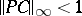.

Internal stability is robust if it is preserved under perturbation of. There are several possible notions of perturbation, typical of which is additive perturbation. So supposeis perturbed to, within. Aboutit is assumed that only a bound on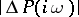is known, namely,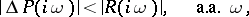where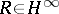. J.C. Doyle and G. Stein [a6] showed that internal stability is preserved under all such perturbations if and only if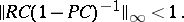(a2)

This leads to the robust stability design problem: Givenand, findso that the feedback system is internally stable and (a2) holds.

The second problem relates to the same feedback system. Suppose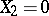,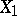represents a disturbance signal, and the objective is to reduce the effect ofon the output. The transfer function fromtoequals. Suppose, in addition, that the disturbance is not a fixed signal, but can be the output of another system with any input inof unit norm; let this latter system have transfer functionin. Then, in view of (a1), the supremal-norm of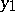over all such disturbances equals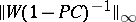. This leads to the disturbance attenuation problem: Givenand, findto achieve internal stability and minimize.

The above two problems are special cases of the more general standardcontrol problem. It can be solved by reduction to the Nehari problem of approximating a function in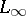(bounded functions on the imaginary axis) by one in. A summary of this theory is in [a7], and a detailed treatment is in [a8].